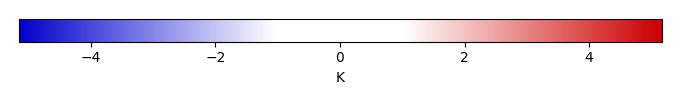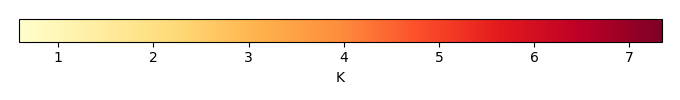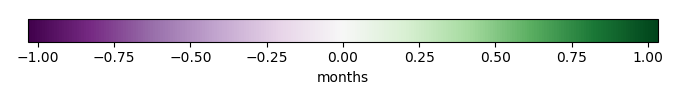# Mean State

Period Mean (original grids) [K]
Model Period Mean (intersection) [K]
Model Period Mean (complement) [K]
Benchmark Period Mean (intersection) [K]
Benchmark Period Mean (complement) [K]
Bias [K]
RMSE [K]
Phase Shift [months]
Bias Score 
RMSE Score 
Seasonal Cycle Score 
Spatial Distribution Score 
Interannual Variability Score 
Overall Score 
Benchmark [-] 304.
CLM5PHSOFF [-] 305. 305. 303. 304. 304. 1.71 2.03 0.343 0.307 0.647 0.955 0.998 0.804 0.726
CLM5PHSON [-] 305. 305. 304. 304. 304. 1.75 2.06 0.325 0.300 0.649 0.958 0.999 0.801 0.726
Period Mean (original grids) [K]
Model Period Mean (intersection) [K]
Model Period Mean (complement) [K]
Benchmark Period Mean (intersection) [K]
Benchmark Period Mean (complement) [K]
Bias [K]
RMSE [K]
Phase Shift [months]
Bias Score 
RMSE Score 
Seasonal Cycle Score 
Spatial Distribution Score 
Interannual Variability Score 
Overall Score 
Benchmark [-] 307.
CLM5PHSOFF [-] 309. 309. 304. 307. 305. 1.94 2.17 0.188 0.499 0.759 0.985 0.997 0.882 0.814
CLM5PHSON [-] 309. 309. 305. 307. 305. 1.99 2.21 0.171 0.491 0.768 0.986 0.997 0.882 0.815
Period Mean (original grids) [K]
Model Period Mean (intersection) [K]
Model Period Mean (complement) [K]
Benchmark Period Mean (intersection) [K]
Benchmark Period Mean (complement) [K]
Bias [K]
RMSE [K]
Phase Shift [months]
Bias Score 
RMSE Score 
Seasonal Cycle Score 
Spatial Distribution Score 
Interannual Variability Score 
Overall Score 
Benchmark [-] 301.
CLM5PHSOFF [-] 303. 303. 299. 301. 302. 1.74 2.06 0.324 0.445 0.724 0.961 0.998 0.835 0.781
CLM5PHSON [-] 303. 303. 300. 301. 302. 1.80 2.10 0.302 0.436 0.738 0.963 0.998 0.831 0.784
Period Mean (original grids) [K]
Model Period Mean (intersection) [K]
Model Period Mean (complement) [K]
Benchmark Period Mean (intersection) [K]
Benchmark Period Mean (complement) [K]
Bias [K]
RMSE [K]
Phase Shift [months]
Bias Score 
RMSE Score 
Seasonal Cycle Score 
Spatial Distribution Score 
Interannual Variability Score 
Overall Score 
Benchmark [-] 266.
CLM5PHSOFF [-] 267. 266. 270. 266. 269. 0.513 1.77 0.0338 0.943 0.910 0.998 1.00 0.900 0.943
CLM5PHSON [-] 266. 266. 270. 266. 269. 0.589 1.77 0.0318 0.941 0.912 0.998 0.999 0.901 0.944
Period Mean (original grids) [K]
Model Period Mean (intersection) [K]
Model Period Mean (complement) [K]
Benchmark Period Mean (intersection) [K]
Benchmark Period Mean (complement) [K]
Bias [K]
RMSE [K]
Phase Shift [months]
Bias Score 
RMSE Score 
Seasonal Cycle Score 
Spatial Distribution Score 
Interannual Variability Score 
Overall Score 
Benchmark [-] 304.
CLM5PHSOFF [-] 305. 305. 303. 304. 303. 1.21 1.75 1.03 0.177 0.512 0.845 0.907 0.800 0.626
CLM5PHSON [-] 305. 305. 304. 304. 303. 1.33 1.84 1.04 0.163 0.510 0.845 0.900 0.811 0.623
Period Mean (original grids) [K]
Model Period Mean (intersection) [K]
Model Period Mean (complement) [K]
Benchmark Period Mean (intersection) [K]
Benchmark Period Mean (complement) [K]
Bias [K]
RMSE [K]
Phase Shift [months]
Bias Score 
RMSE Score 
Seasonal Cycle Score 
Spatial Distribution Score 
Interannual Variability Score 
Overall Score 
Benchmark [-] 276.
CLM5PHSOFF [-] 276. 276. 278. 276. 278. 0.116 1.14 0.00686 0.963 0.938 1.00 1.00 0.972 0.968
CLM5PHSON [-] 276. 276. 278. 276. 278. 0.272 1.09 0.00657 0.962 0.943 1.00 1.00 0.971 0.970
Period Mean (original grids) [K]
Model Period Mean (intersection) [K]
Model Period Mean (complement) [K]
Benchmark Period Mean (intersection) [K]
Benchmark Period Mean (complement) [K]
Bias [K]
RMSE [K]
Phase Shift [months]
Bias Score 
RMSE Score 
Seasonal Cycle Score 
Spatial Distribution Score 
Interannual Variability Score 
Overall Score 
Benchmark [-] 286.
CLM5PHSOFF [-] 287. 287. 292. 286. 289. 0.925 1.61 0.0541 0.870 0.926 0.996 1.00 0.941 0.943
CLM5PHSON [-] 287. 286. 291. 286. 289. 0.949 1.62 0.0587 0.869 0.927 0.996 1.00 0.942 0.943
Period Mean (original grids) [K]
Model Period Mean (intersection) [K]
Model Period Mean (complement) [K]
Benchmark Period Mean (intersection) [K]
Benchmark Period Mean (complement) [K]
Bias [K]
RMSE [K]
Phase Shift [months]
Bias Score 
RMSE Score 
Seasonal Cycle Score 
Spatial Distribution Score 
Interannual Variability Score 
Overall Score 
Benchmark [-] 302.
CLM5PHSOFF [-] 304. 304. 303. 302. 304. 1.33 1.91 0.195 0.602 0.813 0.980 1.00 0.915 0.854
CLM5PHSON [-] 304. 304. 304. 302. 304. 1.34 1.91 0.200 0.600 0.817 0.980 1.00 0.917 0.855
Period Mean (original grids) [K]
Model Period Mean (intersection) [K]
Model Period Mean (complement) [K]
Benchmark Period Mean (intersection) [K]
Benchmark Period Mean (complement) [K]
Bias [K]
RMSE [K]
Phase Shift [months]
Bias Score 
RMSE Score 
Seasonal Cycle Score 
Spatial Distribution Score 
Interannual Variability Score 
Overall Score 
Benchmark [-] 268.
CLM5PHSOFF [-] 267. 267. 270. 268. 268. -0.191 1.46 0.0126 0.965 0.918 0.999 1.00 0.912 0.952
CLM5PHSON [-] 267. 267. 269. 268. 268. -0.198 1.44 0.0151 0.965 0.919 0.999 1.00 0.912 0.952
Period Mean (original grids) [K]
Model Period Mean (intersection) [K]
Model Period Mean (complement) [K]
Benchmark Period Mean (intersection) [K]
Benchmark Period Mean (complement) [K]
Bias [K]
RMSE [K]
Phase Shift [months]
Bias Score 
RMSE Score 
Seasonal Cycle Score 
Spatial Distribution Score 
Interannual Variability Score 
Overall Score 
Benchmark [-] 301.
CLM5PHSOFF [-] 302. 303. 296. 301. 299. 1.73 1.97 0.124 0.677 0.874 0.991 0.998 0.902 0.886
CLM5PHSON [-] 303. 303. 297. 301. 299. 1.76 1.99 0.114 0.673 0.880 0.991 0.997 0.905 0.888
Period Mean (original grids) [K]
Model Period Mean (intersection) [K]
Model Period Mean (complement) [K]
Benchmark Period Mean (intersection) [K]
Benchmark Period Mean (complement) [K]
Bias [K]
RMSE [K]
Phase Shift [months]
Bias Score 
RMSE Score 
Seasonal Cycle Score 
Spatial Distribution Score 
Interannual Variability Score 
Overall Score 
Benchmark [-] 286.
CLM5PHSOFF [-] 287. 286. 287. 286. 289. 0.538 1.30 0.126 0.879 0.903 0.991 0.997 0.940 0.936
CLM5PHSON [-] 286. 286. 287. 286. 289. 0.520 1.28 0.193 0.881 0.906 0.987 0.997 0.941 0.936
Period Mean (original grids) [K]
Model Period Mean (intersection) [K]
Model Period Mean (complement) [K]
Benchmark Period Mean (intersection) [K]
Benchmark Period Mean (complement) [K]
Bias [K]
RMSE [K]
Phase Shift [months]
Bias Score 
RMSE Score 
Seasonal Cycle Score 
Spatial Distribution Score 
Interannual Variability Score 
Overall Score 
Benchmark [-] 292.
CLM5PHSOFF [-] 289. 293. 264. 292. 295. 1.11 1.81 0.166 0.691 0.836 0.981 0.997 0.909 0.875
CLM5PHSON [-] 289. 293. 252. 292. 295. 1.15 1.82 0.166 0.687 0.840 0.981 0.997 0.910 0.876
Period Mean (original grids) [K]
Model Period Mean (intersection) [K]
Model Period Mean (complement) [K]
Benchmark Period Mean (intersection) [K]
Benchmark Period Mean (complement) [K]
Bias [K]
RMSE [K]
Phase Shift [months]
Bias Score 
RMSE Score 
Seasonal Cycle Score 
Spatial Distribution Score 
Interannual Variability Score 
Overall Score 
Benchmark [-] 303.
CLM5PHSOFF [-] 304. 304. 299. 303. 303. 1.70 2.08 0.115 0.737 0.893 0.991 0.998 0.913 0.904
CLM5PHSON [-] 304. 304. 301. 303. 303. 1.71 2.09 0.115 0.736 0.892 0.991 0.998 0.913 0.904
Period Mean (original grids) [K]
Model Period Mean (intersection) [K]
Model Period Mean (complement) [K]
Benchmark Period Mean (intersection) [K]
Benchmark Period Mean (complement) [K]
Bias [K]
RMSE [K]
Phase Shift [months]
Bias Score 
RMSE Score 
Seasonal Cycle Score 
Spatial Distribution Score 
Interannual Variability Score 
Overall Score 
Benchmark [-] 268.
CLM5PHSOFF [-] 269. 269. 269. 268. 270. 0.331 1.58 0.0173 0.940 0.903 0.999 1.00 0.911 0.943
CLM5PHSON [-] 269. 269. 268. 268. 270. 0.359 1.57 0.0160 0.940 0.903 0.999 0.999 0.912 0.943
Period Mean (original grids) [K]
Model Period Mean (intersection) [K]
Model Period Mean (complement) [K]
Benchmark Period Mean (intersection) [K]
Benchmark Period Mean (complement) [K]
Bias [K]
RMSE [K]
Phase Shift [months]
Bias Score 
RMSE Score 
Seasonal Cycle Score 
Spatial Distribution Score 
Interannual Variability Score 
Overall Score 
Benchmark [-] 302.
CLM5PHSOFF [-] 303. 303. 302. 302. 303. 1.34 2.03 0.272 0.539 0.744 0.974 0.988 0.908 0.816
CLM5PHSON [-] 303. 303. 303. 302. 303. 1.39 2.06 0.284 0.533 0.747 0.973 0.988 0.908 0.816
Period Mean (original grids) [K]
Model Period Mean (intersection) [K]
Model Period Mean (complement) [K]
Benchmark Period Mean (intersection) [K]
Benchmark Period Mean (complement) [K]
Bias [K]
RMSE [K]
Phase Shift [months]
Bias Score 
RMSE Score 
Seasonal Cycle Score 
Spatial Distribution Score 
Interannual Variability Score 
Overall Score 
Benchmark [-] 292.
CLM5PHSOFF [-] 293. 293. 297. 292. 297. 0.958 1.27 0.0733 0.883 0.934 0.995 0.997 0.965 0.951
CLM5PHSON [-] 293. 293. 299. 292. 297. 0.973 1.27 0.0812 0.882 0.935 0.995 0.997 0.964 0.951
Period Mean (original grids) [K]
Model Period Mean (intersection) [K]
Model Period Mean (complement) [K]
Benchmark Period Mean (intersection) [K]
Benchmark Period Mean (complement) [K]
Bias [K]
RMSE [K]
Phase Shift [months]
Bias Score 
RMSE Score 
Seasonal Cycle Score 
Spatial Distribution Score 
Interannual Variability Score 
Overall Score 
Benchmark [-] 279.
CLM5PHSOFF [-] 280. 280. 282. 279. 282. 0.510 1.20 0.0402 0.932 0.924 0.997 0.999 0.958 0.956
CLM5PHSON [-] 280. 280. 281. 279. 282. 0.601 1.19 0.0353 0.928 0.928 0.998 0.999 0.959 0.956
Period Mean (original grids) [K]
Model Period Mean (intersection) [K]
Model Period Mean (complement) [K]
Benchmark Period Mean (intersection) [K]
Benchmark Period Mean (complement) [K]
Bias [K]
RMSE [K]
Phase Shift [months]
Bias Score 
RMSE Score 
Seasonal Cycle Score 
Spatial Distribution Score 
Interannual Variability Score 
Overall Score 
Benchmark [-] 291.
CLM5PHSOFF [-] 292. 292. 291. 291. 292. 1.08 1.69 0.0460 0.853 0.904 0.997 0.999 0.941 0.933
CLM5PHSON [-] 292. 292. 293. 291. 292. 1.18 1.71 0.0437 0.849 0.908 0.997 0.999 0.942 0.934
Period Mean (original grids) [K]
Model Period Mean (intersection) [K]
Model Period Mean (complement) [K]
Benchmark Period Mean (intersection) [K]
Benchmark Period Mean (complement) [K]
Bias [K]
RMSE [K]
Phase Shift [months]
Bias Score 
RMSE Score 
Seasonal Cycle Score 
Spatial Distribution Score 
Interannual Variability Score 
Overall Score 
Benchmark [-] 304.
CLM5PHSOFF [-] 305. 306. 303. 304. 304. 1.66 1.96 0.380 0.243 0.645 0.948 1.00 0.897 0.730
CLM5PHSON [-] 306. 306. 304. 304. 304. 1.72 2.01 0.388 0.233 0.648 0.948 1.00 0.903 0.730
Period Mean (original grids) [K]
Model Period Mean (intersection) [K]
Model Period Mean (complement) [K]
Benchmark Period Mean (intersection) [K]
Benchmark Period Mean (complement) [K]
Bias [K]
RMSE [K]
Phase Shift [months]
Bias Score 
RMSE Score 
Seasonal Cycle Score 
Spatial Distribution Score 
Interannual Variability Score 
Overall Score 
Benchmark [-] 301.
CLM5PHSOFF [-] 303. 303. 299. 301. 297. 1.67 1.83 0.308 0.491 0.770 0.961 0.999 0.925 0.819
CLM5PHSON [-] 303. 303. 300. 301. 297. 1.72 1.88 0.284 0.481 0.782 0.964 0.999 0.926 0.822
Period Mean (original grids) [K]
Model Period Mean (intersection) [K]
Model Period Mean (complement) [K]
Benchmark Period Mean (intersection) [K]
Benchmark Period Mean (complement) [K]
Bias [K]
RMSE [K]
Phase Shift [months]
Bias Score 
RMSE Score 
Seasonal Cycle Score 
Spatial Distribution Score 
Interannual Variability Score 
Overall Score 
Benchmark [-] 290.
CLM5PHSOFF [-] 292. 292. 291. 290. 289. 1.66 2.37 0.257 0.557 0.762 0.966 0.996 0.916 0.826
CLM5PHSON [-] 292. 292. 293. 290. 289. 1.69 2.38 0.285 0.555 0.763 0.963 0.995 0.916 0.826

# Temporally integrated period mean

BENCHMARK MEANMODEL MEANBIASBIAS SCORERMSERMSE SCOREBENCHMARK INTERANNUAL VARIABILITYMODEL INTERANNUAL VARIABILITYINTERANNUAL VARIABILITY SCOREBENCHMARK MAX MONTHMODEL MAX MONTHDIFFERENCE IN MAX MONTHSEASONAL CYCLE SCORESPATIAL TAYLOR DIAGRAMMODEL COLORS# Spatially integrated regional mean

MODEL COLORSREGIONAL MEANANNUAL CYCLEMONTHLY ANOMALYANNUAL CYCLE# All Models

BenchmarkCLM5PHSOFFCLM5PHSON# Data Information

creation_date: Fri Feb 8 14:12:14 PST 2019

history: 2018-01-15: download from https://crudata.uea.ac.uk/cru/data/hrg/cru_ts_4.01/cruts.1709081022.v4.01/ 2019-02-06: converted and created to netCDF4 for ILAMB package

original_resolution: 0.5x0.5

resolution: 0.5x0.5

original_frequency: mon

frequency: mon

references: Harris et al., 2014, Int. J. Climatol., doi:10.1002/joc.3711

institution: Original Data held at British Atmospheric Data Centre, RAL, UK.

source: Run ID = 1709081022. Data generated from:TMX derived from gridded TMP & DTR absolutes, DTR in turn derived from tmn.1708171135.dtb, tmx.1708171215.dtb

title: CRU TS4.01 Maximum Temperature

Conventions: CF-1.7